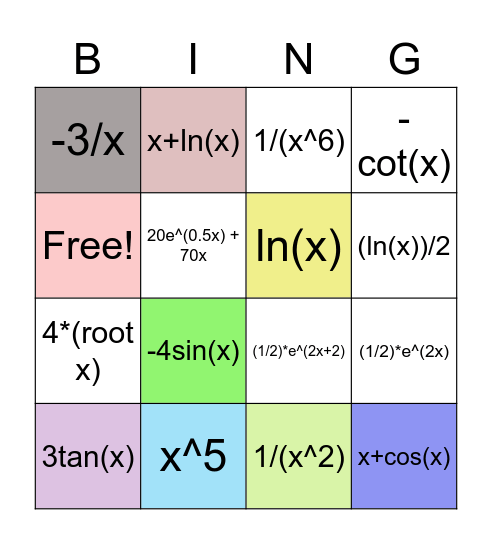# Anti-Derivatives!This bingo card has 16 words: x^5, (1/2)*e^(2x), 1/(x^6), -3/x, 4*(root x), x+ln(x), (1/2)*e^(2x+2), 1/(x^2), Free!, 20e^(0.5x) + 70x, 3tan(x), -cot(x), -4sin(x), (ln(x))/2, ln(x) and x+cos(x).

## Play Online